# MA005 Calculus HELP NEEDED

#1

At what point should the engine be turned off in order to coast along the tangent line to a base at (1,3)?
This doesn’t exist because it is inside the parabola. But, how do I find that out?

#2

Also, how does one find f(x) given f’(x)? This is questions 32-37 in MA005 3.2, but I don’t see how to solve this?

#4

Step 1) Find the slope of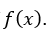.
Step 2) Consider the points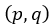and find the value of the slope at that point.
Step 3) Find equation of the tangent line using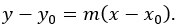.
Step 4) Find equation of the tangent at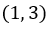and solve for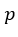.

Step 1: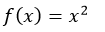Slope is given by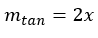Step 2
Let us assume that the engine is turned off at the pointon the curve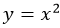.
As per given conditionmust have go to the tangent line to the curve at point.
The pointis on the curve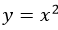, so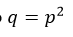The point will be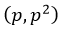.
Slope of the tangent line is given by,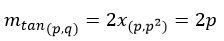Step 3:
Equation of the tangent line at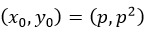is given by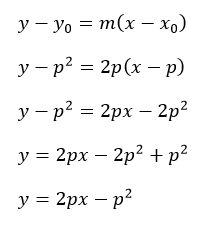Equation of the tangent line at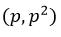is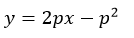Step 4:
Equation of the tangent line atisEquation of the tangent line atis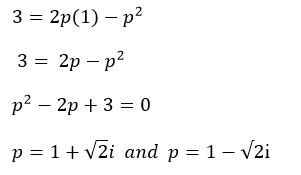The value ofare not real, so no real point is exist which passed through point.
Conclusion:
There is no any point exist such that the engine turned off in order to coast along the tangent line at point.

#5

Thank you! Now I understand.